# Civil Engineering - UPSC Civil Service Exam Questions

16.

When is a masonry wall known as a shear wall ?

 A. If the earthquake load is out-of-plane B. If the earthquake load is in-plane C. If it is under reinforced D. If it is placed as infill to the frame

Explanation:

No answer description available for this question. Let us discuss.

17.

A parabolic arch, symmetrical, with hinges at centre and ends, carries a point load P at distance x from left support. The arch has a span of 20 m and rise of 5 m. What is the value of x if the left hinge reaction is inclined with a slope of two vertical on one horizontal ?

 A. 8 m B. 5 m C. 4 m D. 2.5 m

Explanation:

No answer description available for this question. Let us discuss.

18.

Which of the following equations will be satisfied by irrotational flow of an incompressible fluid?

 A.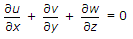B.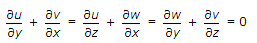C.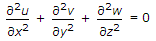D.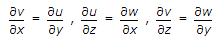Explanation:

No answer description available for this question. Let us discuss.

19.

Consider the following statements for the column with a bracket as shown in the figure :
1. Shear force is constant throughout.
2. Maximum moment in the column is P.e.
3. The compressive axial force in the column is 0.4. p.
Which of the statements given above is/are correct ?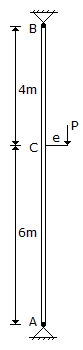A. 1, 2 and 3 B. 1 only C. 1 and 3 D. 2 only

Explanation:

No answer description available for this question. Let us discuss.

20.

Increase in fineness of cement

 A. reduces the rate of strength development and leads to higher shrinkage B. increases the rate of strength development and reduces the rate of deterioration C. decreases the rate of strength development and increases the bleeding of cement D. increases the rate of strength development and leads to higher shrinkage